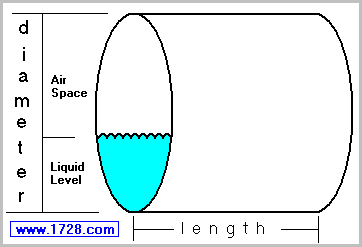Horizontal Cylindrical Tank Calculator and Dipstick Chart To see how this volume is calculated, click here. Scroll Down for Instructions>>>>>
>>>>>
>>>>>

The numbers input above are in which units?
or or or

Dipstick Increments
For a "printer-friendly" chart

 I n s t r u c t i o n s Use this calculator for computing the volume of partially-filled horizontal cylinders. If you need a vertical cylinder calculator, click here. Besides calculating volume for any particular depth, this calculator can also produce a "dipstick chart" showing volume across the entire range of tank depths.
 Example: Inputting liquid level = 3, diameter = 24, tank length = 30, then clicking "Inches" will display the total tank volume in cubic inches and US Gallons and will also show the volume at the 3 inch level. In addition, a dipstick chart is automatically generated. The default dipstick chart is in increments of one but you can change that by clicking on one of the increment buttons. An alternative to this would be inputting a different set of units. For example, if you are inputting information for a tank that is 1.25 feet in diameter and 2 feet in length, you will end up with a default dipstick chart that has two lines - zero feet and one foot. It would be better to input this as 15 inches and 24 inches, then clicking the "inches" button so that the default chart now has 15 lines. The default dipstick chart increments are based on the diameter value.

 Significant Figures >>> Numbers are displayed in scientific notation with the amount of significant figures you specify. For easier readability, numbers between .001 and 1,000 will not be in scientific notation but will still have the same precision. You may change the number of significant figures displayed by changing the number in the box above. Most browsers, will display the answers properly but if you are seeing no answers at all, enter a zero in the box above, which will eliminate all formatting but at least you will see the answers.